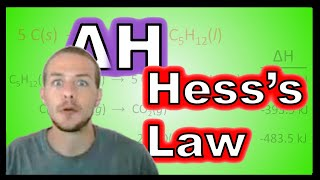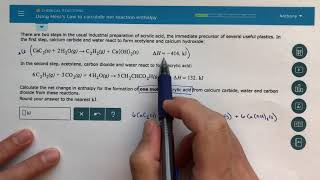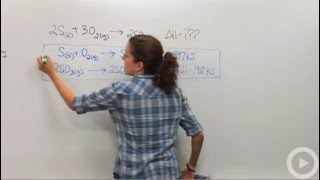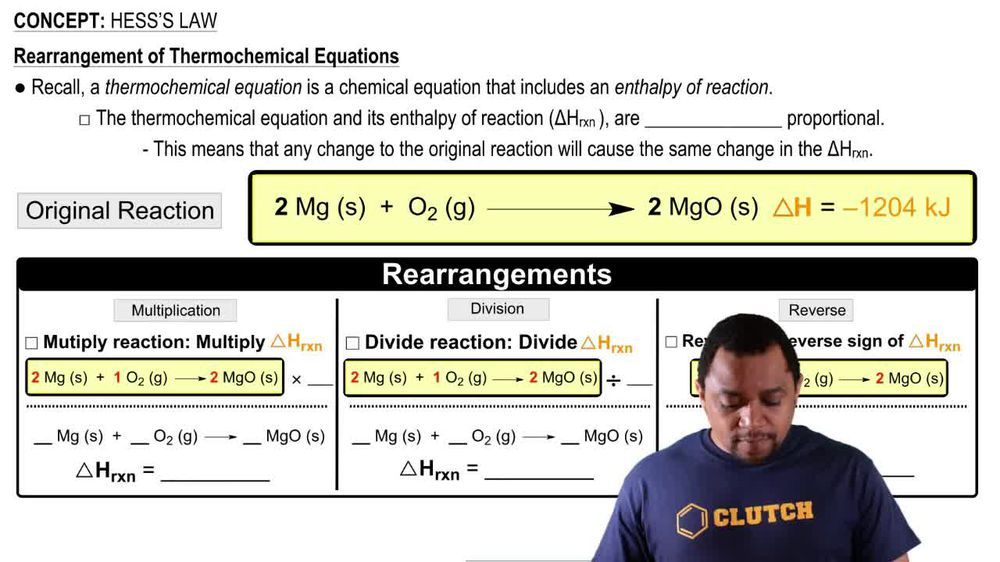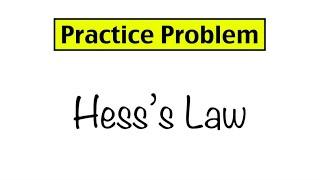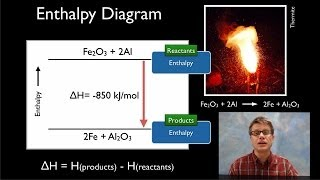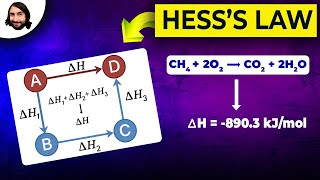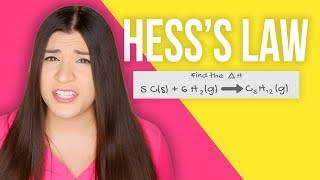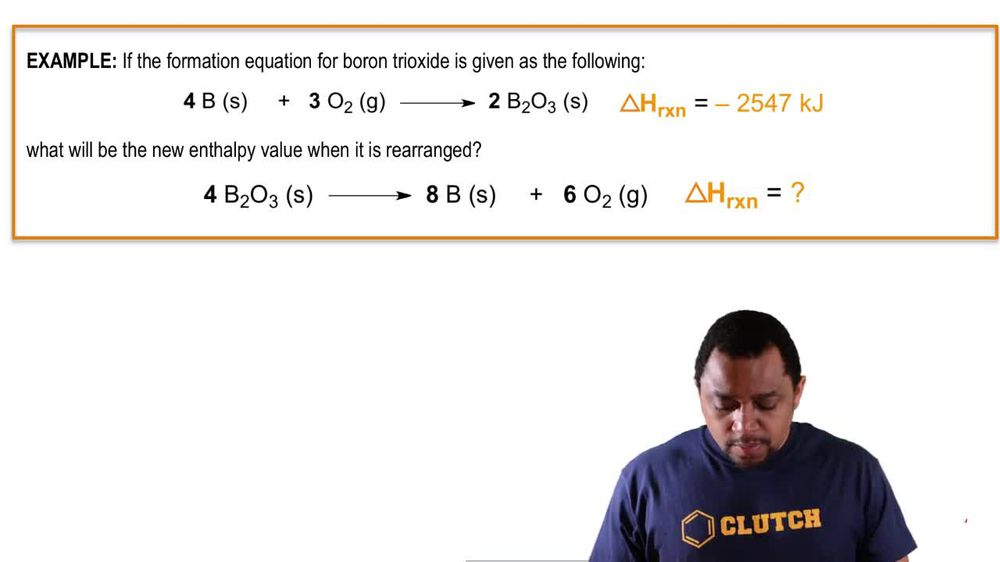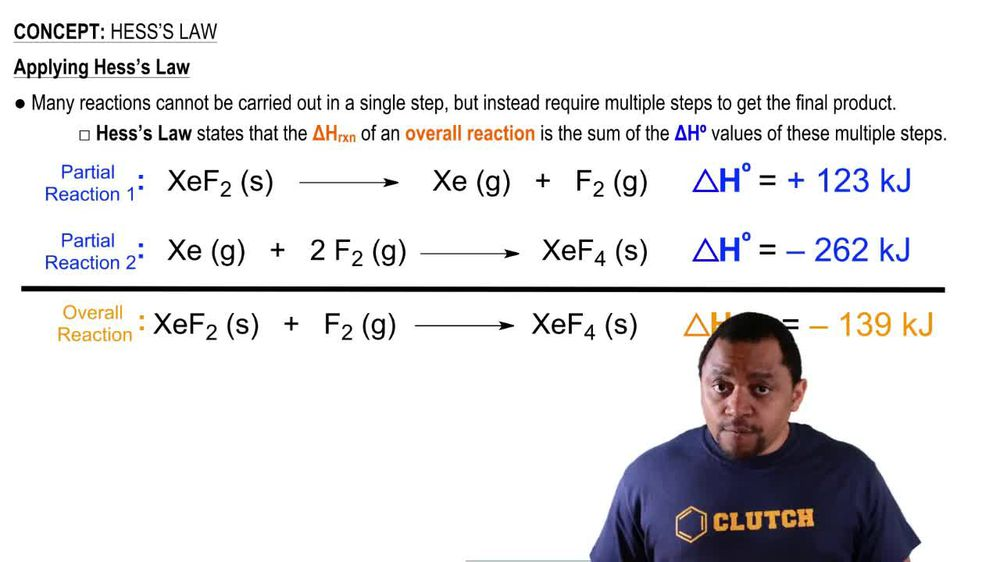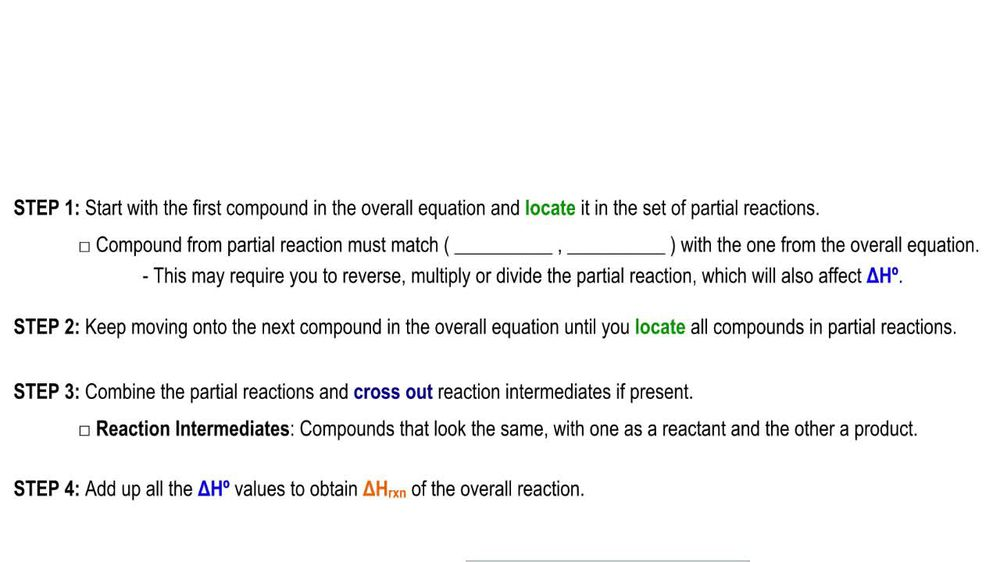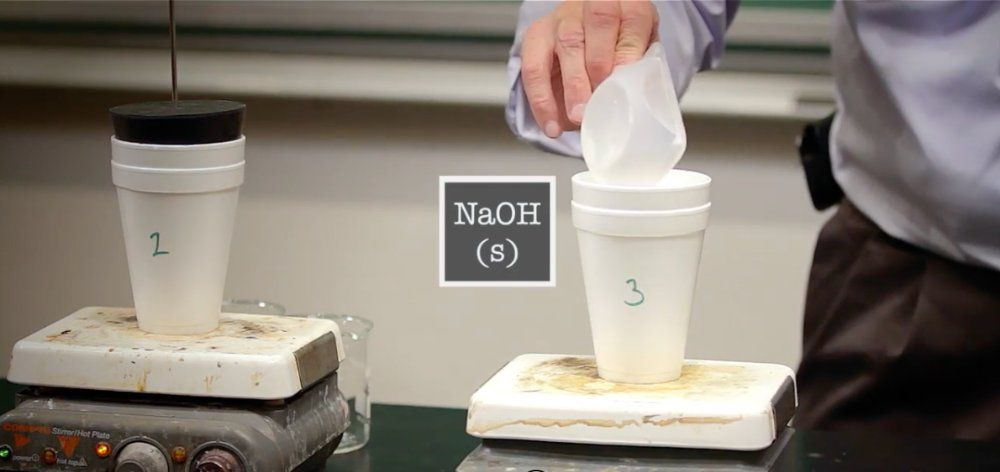Start typing, then use the up and down arrows to select an option from the list.
1. 8. Thermochemistry2. Hess's Law# Hess's Law Example 1

by Jules Bruno
197 views
1
1
in this example question. It says, if the formation equation for boron trioxide is given as the following four moles of Be solid plus three moles of +02 gas produces two moles off boron trioxide Solid has an entropy of negative 25 47 killing jewels. What will be the new entropy value when it's rearranged? All right, so we have to see what the changes that the original equation under wind to determine the new Delta h Well, we can see one thing that happened is what was a product is now a reactant and what was a reactant are now products. So we reversed it. Yeah, the next thing we should notice are the coefficients here it was a two, but now it's a four. This was a four, but now it's an eight. This was a three banal to six, so we didn't Onley reverse the reaction, but we multiplied by two. Yeah, Now, remember, when you reverse the reaction that's going to reverse the sign of Delta H. So now it's gonna be positive and whatever number I multiply my equation by, I multiply Delta H by that same number. So you multiply this by two. When you do that, it gives you 50 94 killer jewels. So for my new equation would be a positive 5000 and killer jewels. Reversing the reaction reverses the sign. Multiplying it by a value means I multiply my delta h value by that same number.